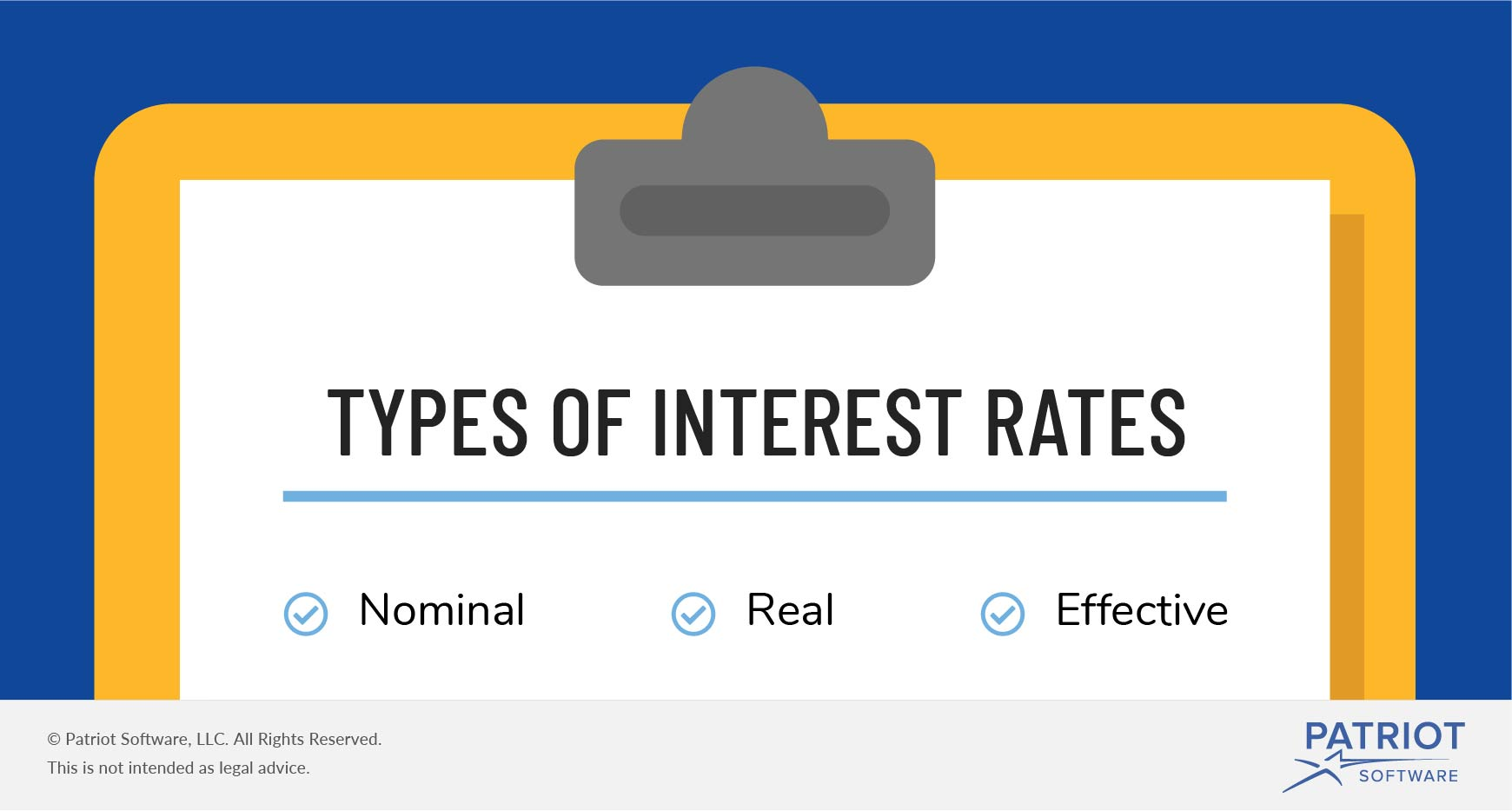What Is Interest? | Examples, Types, & More

# What Is Interest?

As a business owner, you likely borrow money to help fund your startup. And when you need a business loan, lenders typically charge you interest. So, what is interest?

## What does interest mean?

Interest is the rate lenders charge individuals to borrow money. Essentially, interest is the cost of borrowing money. The amount you borrow is typically referred to as the principal amount.

How does interest work? When you borrow money from a lender, you pay interest. Likewise, if you lend money, you earn interest. Common types of loans that have interest include student loans, business loans, and loans for mortgages.

If you need a loan for a new business, you might apply for business loans. Or, you may opt to use a personal loan for business.

The lender can charge a fixed percentage, known as an interest rate. Or, interest can be a flat amount or fee. The interest rate determines the amount of interest the borrower must pay.

The amount the lender charges for interest depends on many factors. Some things that can impact interest rates include:

• Type of loan
• Amount of expected inflation
• Length of time money is lent
• Liquidity of the loan
• Government intervention on interest rates
• Credit history

Usually, interest rates are set as an annual percentage. However, time periods for interest can be longer or shorter than a year.

### Simple and compound interest

Lenders can apply two kinds of interest to loans: simple and compound. Business loan rates use either simple or compound interest.

Simple interest is a set rate on the principal amount lent to the borrower. Compound interest refers to the interest on the principal amount and the compounding interest paid.

Whether or not an interest rate is simple or compound depends on the type of interest rate.

## Types of interest rates

There are several ways to determine the amount of interest on a loan. Interest rate amounts can be as simple as setting a flat percent (e.g., seven percent). Or, lenders might factor in credit and other small business debt to determine an interest rate formula.

There are three main types of interest rates, including nominal, real, and effective interest.### Nominal

Nominal interest refers to interest that computes from the principal amount. The rate provides the exact amount of interest a person earns or pays for a loan.

For example, a loan of \$100 with a nominal interest rate of 6% would accrue \$6 in interest (\$100 X 0.06).

The rate does not change if the amount of the loan increases. A borrower would still pay 6% if the loan increased to \$1,000.

### Real

Real interest is similar to nominal interest. Both are considered types of simple interest. However, real interest factors in inflation. Real interest takes into consideration that the purchasing power of a lender can change due to inflation.

To calculate real interest, take the nominal interest rate and subtract the rate of inflation.

Nominal interest rate – Inflation = Real interest rate

For example, say your nominal interest rate is 7% and the inflation rate is 4%. Your real interest rate is 3%.

### Effective

Effective interest uses compounding. When calculating effective interest rates, include the principal as well as the amount earned from previous interest.

Effective interest allows lenders to earn more since the interest factors in the principal amount and accrued interest.

For example, say you borrow \$1,000 for seven years at a 10% interest rate. During the first year, your interest would be \$100. The next year, your interest amount would include the principal amount plus interest, which is \$1,100. This means your interest in the second year would equal \$110 (\$1,100 x 0.10).

Do you need a way to keep track of your small business funds? Patriot’s online accounting software lets you easily monitor your cash flow and business loans. And, we offer free, U.S.-based support. Try it for free today!

This article has been updated from its original publication date of March 26, 2015.